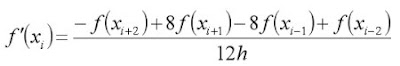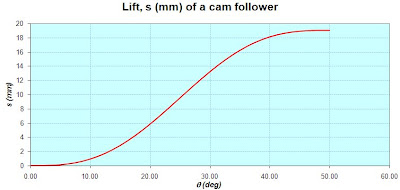Improve math skills of your kids - Learn step-by-step arithmetic from Math games

Math: Unknown - Step-by-step math calculation game for iOS.

Math: Unknown is much more than a math game. It is a step-by-step math calculation game which will teach users how to calculate in the correct order rather than just asking only the final calculated results.

The app consists of four basic arithmetic operations which are addition, subtraction, multiplication and division. In order to get started, users who are new to arithmetic can learn from animated calculation guides showing step-by-step procedures of solving each type of operation. It is also helpful for experienced users as a quick reference.

Generally, addition and subtraction may be difficult for users who just start learning math especially when questions require carrying or borrowing (also called regrouping). The app helps users to visualize the process of carrying and borrowing in the way it will be done on paper. Once users understand how these operations work, they are ready to learn multiplication and division.

For most students, division is considered as the most difficult arithmetic operation to solve. It is a common area of struggle since it requires prior knowledge of both multiplication and subtraction. To help users understand division, the app uses long division to teach all calculation procedures. Relevant multiplication table will be shown beside the question. Users will have to pick a number from the table which go into the dividend. Multiplication of selected number and divisor is automatically calculated, but the users have to do subtraction and drop down the next digit themselves. Learning whole calculation processes will make them master it in no time.

Math: Unknown is a helpful app for students who seriously want to improve arithmetic calculation skills.

Numerical Methods - First derivative using Excel formula

Numerical methods can be very helpful for calculation of mechanical engineering design. What I would like to introduce in this post is about calculation of the first derivative using numerical methods in Microsoft Excel without using any math software.

From the textbook, there are a lot of formula and explanation about how we get this formula. So I would skip that part and directly start with the selected formula as an example.

We know that the first derivative of function f(x) can be calculated from the following equationIt is the formula to calculate first derivative using interior points that give the error of h4
That means we will use the values of function at other 4 points around the center of interest to calculate the first derivative and the smaller the step size (h), the higher accuracy of calculation result.

The following is the equation of favorite cam curve - CycloidThe cam follower will slowly move at the beginning of time and start accelerating, then decelerating in the middle of time and slowly stops at the end of movement. This give smooth motion of cam mechanism.We can calculate the velocity of the cam follower using the following formula.What we have to do is to make a table in excel and enter the formula of f(x), for this case, it's the cycloid motion function.The above table is calculated using 1.25 deg for each step. But the real value of h should be calculated from 1.25 x Pi/180 = 0.021816616 rad.

Then we can calculate the velocity at each point using the formula explained above.
Example: to calculate the first derivative of cycloid motion at 15 deg
If camshaft speed = 50 rpm, then omega = 2 x Pi x 50/60 = 5.235987756 rad/s

Then the velocity at 15 deg can be calculated fromf'(15 deg) = [-4.20358 + 8x3.48065 - 8x2.23829 + 1.72606]/(12x0.021816616) x 5.235987756 = 149.2272246 mm/s = 0.1492 m/s

If we do the same calculation for the remaining points, we can then get the velocity profile of cycloid motion. Try it yourself and get the result as follows,No need to use any math software. Excel can easily help you calculate first derivative using knowledge of numerical methods.leoo said…
So how do you calculate for the derivative for points i = 1 and i = 2? Since the formula requires values for f(x at i-1) and f(x at i-2).

Hoping for your reply asap. Hehe. I'm finishing my lab report for tomorrow.Suparerg Suksai said…
Hi leoo,

I would extend the table and calculate f(x at i-1) and f(x at i-2) like this...

f'(0 deg) = [-f(2.5 deg) + 8xf(1.25 deg) - 8xf(-1.25 deg) + f(-2.5 deg)]/(12x0.021816616) x 5.235987756 = 0.00230668 mm/s

also for f'(1 deg) can be calculated from
f'(1.25 deg) = [-f(3.75 deg) + 8xf(2.5 deg) - 8xf(0 deg) + f(-1.25 deg)]/(12x0.021816616) x 5.235987756 = 1.405807453 mm/s

We can compare this approximated values with the exact solution.

f'(theta) = hm/bm[1 - cos(2*pi*theta/bm)] x omega

from this formula we get
f'(0 deg) = 0 mm/s
f'(1.25 deg) = 1.403529172 mm/s

Ake